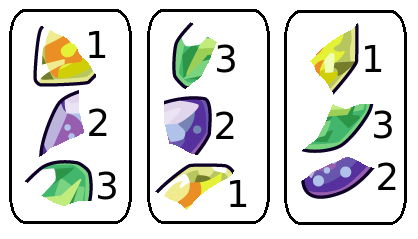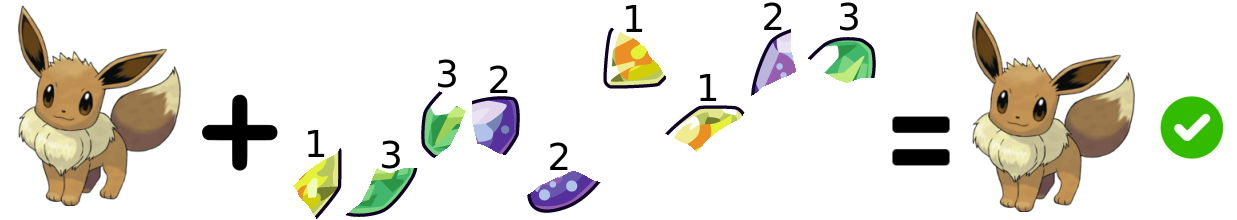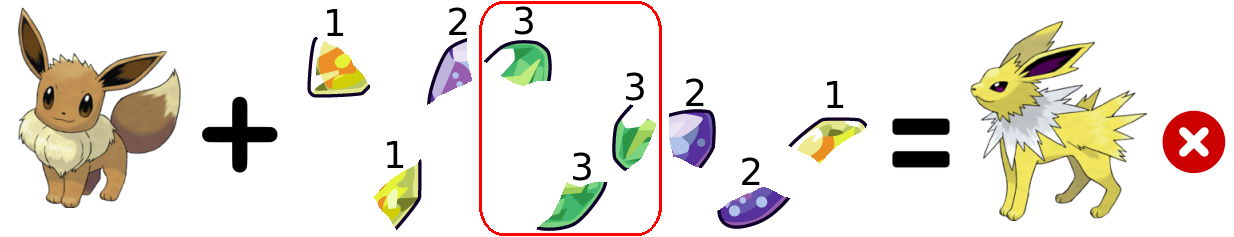시간 제한 메모리 제한 제출 정답 맞은 사람 정답 비율
5 초 512 MB 4 4 4 100.000%

## 문제

As you may know, Eevee can evolve in various ways. For instance, it will evolve when it comes close to one of the evolution stones.

Let’s assume that there are n different evolution stones, numbered with integers from 1 to n. Each of them was split into k fragments. Eevee has grouped all the fragments into k stacks, numbered with integers from 1 to k. Each stack contains exactly n fragments, each of which comes from a different stone. Therefore, we can consider each stack as a permutation of the fragments of the different stones.

Eevee will perform the following operation k · n times: pick one of the non-empty stacks, remove its topmost fragment and add it to the end of a sequence of fragments (which is initially empty). Two ways of combining the stacks into one sequence are considered distinct if at any step we choose a stack with a different index. If after this process there are k neighboring fragments of the same stone in the sequence, Eevee can accidentally evolve. As he is the cutest without any evolutions, we call some way to combine the stacks good if there is no interval of length k containing only the fragments of the same stone.

Let $f(i, j)$ denote the number of good ways to combine the stacks with indices from the range [i, j]. Here, when we consider some interval of length ℓ, we assume that Eevee performs the operation only ℓ · n times and that we prohibit any interval of length ℓ from containing only the fragments of the same stone.

$\left(\sum_{i=1}^{k}\sum_{j=i+1}^{k}f(i,j)\right) \mod{(10^9+7)}\text{.}$

However, it turned out that Eevee shuffled each stack before the process! Therefore, each stack contains a random permutation of the fragments. Each of the input permutations is chosen equiprobably from all n! possible permutations and independently from each other.

## 입력

The first line contains two integers k and n (2 ≤ k, n ≤ 300) — the number of stacks and the number of fragments in each stack.

Each of the next k lines contains n integers. The j-th number in the i-th line is ai,j (1 ≤ ai,j ≤ n, ai,j ≠ ai,l for j ≠ l) and denotes the j-th number from the top in the i-th stack.

## 출력

Output the value of the sum described in the problem statement.

## 예제 입력 1

3 3
1 2 3
3 2 1
1 3 2


## 예제 출력 1

1464


## 예제 입력 2

4 2
1 2
2 1
1 2
2 1


## 예제 출력 2

2466


## 힌트

Consider calculating f(1, 3) in the first sample. Eevee has three stacks of the fragments of the stones:One of the good ways to combine them is as follows:The following one isn’t good as it contains three 3s in a row:In the first sample f(1, 2) = 8, f(1, 3) = 1446 and f(2, 3) = 10.

In the second sample f(1, 2) = 2, f(1, 3) = 66, f(1, 4) = 2328, f(2, 3) = 2, f(2, 4) = 66 and f(3, 4) = 2.